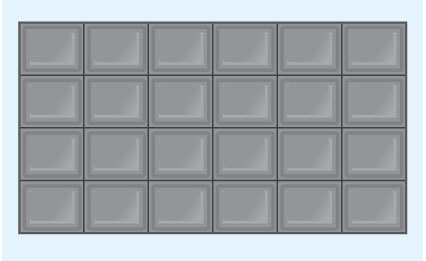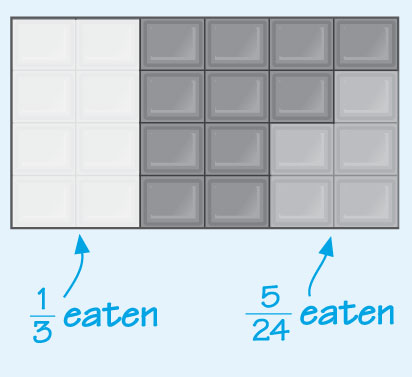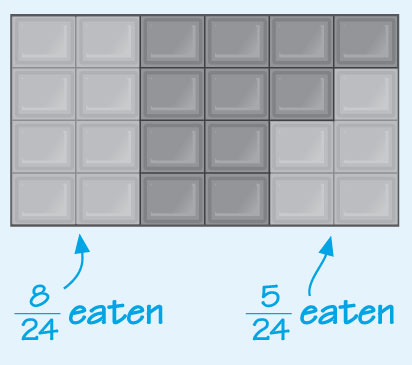Succeed with maths – Part 1

Start this free course now. Just create an account and sign in. Enrol and complete the course for a free statement of participation or digital badge if available.

Free course

# 1 Adding and subtracting fractions

In this section, you will look at how to add and subtract numbers that include fractions. To get started you’ll look at visual representation to help you understand the process used.

The chocolate bar in Figure 1 has 24 pieces. Each piece is 1 out of 24 or of the bar, so the whole bar is , or 1. There are four identical rows, so each row is of the bar.

There are also six identical columns, so each column is of the bar.Figure _unit5.1.1 Figure 1 A chocolate bar

Imagine that you eat eight of the 24 pieces.

That is equivalent to one third of the chocolate bar, because .

You can visualise this by covering up two of the columns. You are still feeling hungry five minutes later, so you decide to eat another five pieces. What fraction of the bar will you have eaten in total? What fraction of the bar is left? Take a look at Figure 2 to help.Figure _unit5.1.2 Figure 2 How much has been eaten?

The fraction eaten will be , but it’s not easy to quickly determine what fraction this is because the two fractions are out of different numbers of parts.

However, if you recall that eating of the bar is the same as eating , the fraction eaten is .

You can check this answer on the bar of chocolate: just count the number of squares that have been eaten.Figure _unit5.1.3 Figure 3 How much has been eaten?

The fraction that is left will be one whole bar with removed from it, or as written in maths language:

So, thinking of the whole bar as , the calculation becomes .

You can check this by counting, again, the number of squares that are left. This method of changing the fractions into the same kind (using equivalent fractions) can be used for adding and subtracting fractions in general. If you want a reminder of equivalent fractions, return to Week 3.

If you need to add or subtract fractions, the first question you need to ask yourself is, ‘Are these the same kind of fractions?’ That is, are they divided into the same number of parts? Or in maths language, are the denominators the same? If the answer is no, then you need to convert the fractions into equivalent fractions with the same denominator in order to add or subtract them.